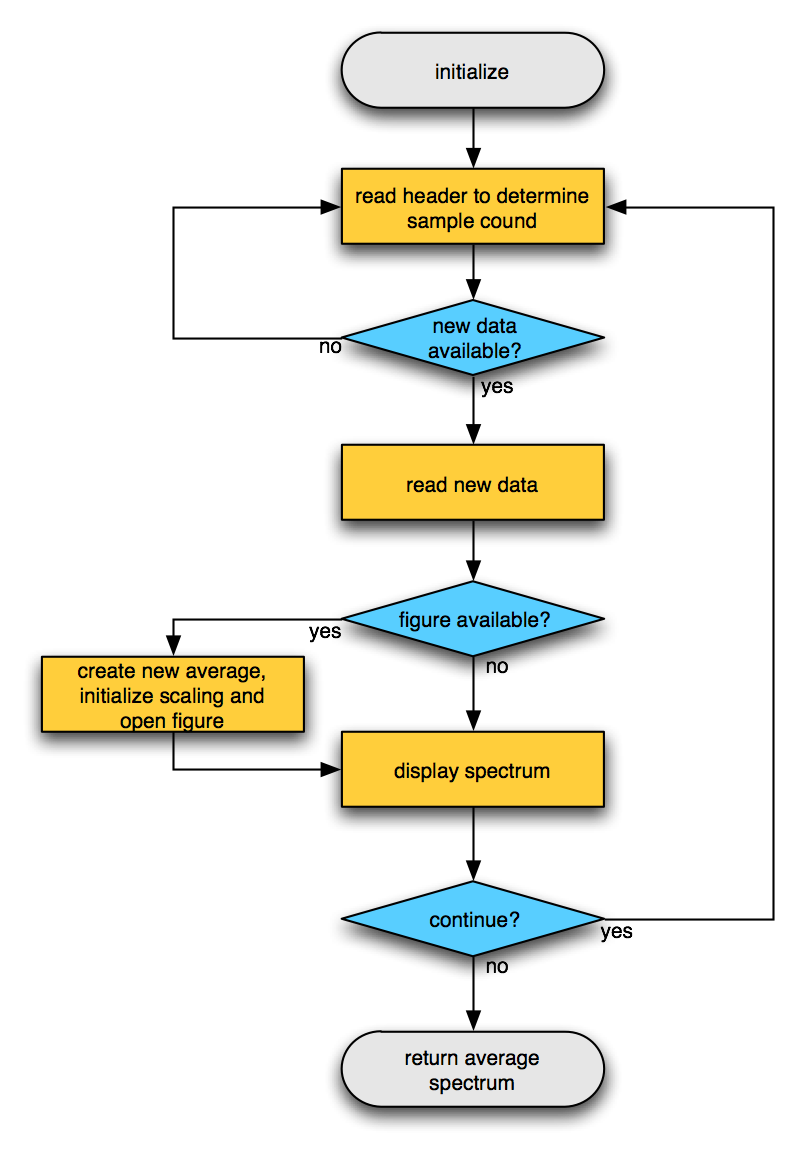Tags: example realtime

# Example real-time power estimate

The ft_realtime_powerestimate function reads data in small chunks and performs a spectral estimation for each chunck. The output of this function is a constantly updating figure with the power spectrum, averaged over the selected channels.

## Flowchart## Example use

The easiest way to try out the ft_realtime_powerestimate example is by starting two MATLAB sessions. In the first session you create some random signal and write it to the buffer by means of *ft_realtime_signalproxy

``````cfg                = [];
cfg.channel        = 1:10;                         % list with channel "names"
cfg.blocksize      = 1;                            % seconds
cfg.fsample        = 250;                          % sampling frequency, Hz
cfg.lpfilter       = 'yes';                        % apply a low-pass filter
cfg.lpfreq         = 20;                           % filter frequency, Hz
cfg.target.dataset = 'buffer://localhost:1972';    % where to write the data
ft_realtime_signalproxy(cfg)
``````

In the second MATLAB session you start the ft_realtime_powerestimate and point it to the buffe

``````cfg                = [];
cfg.blocksize      = 1;                            % seconds
cfg.foilim         = [0 30];                       % frequency-of-interest limits, Hz
cfg.dataset        = 'buffer://localhost:1972';    % where to read the data from
ft_realtime_powerestimate(cfg)
``````

After starting the ft_realtime_powerestimate, you should see a figure that updates itself every second. That figure contains the powerspectrum of the simulated random number signal. If you close the figure, the figure will re-appear and start all over again with the automatic scaling of the vertical axis.

You can also start the two MATLAB sessions on two different computers, where on the second you would then point the reading function to the first computer.

## MATLAB code

``````function ft_realtime_powerestimate(cfg)

% FT_REALTIME_POWERESTIMATE is an example realtime application for online
% power estimation. It should work both for EEG and MEG.
%
% Use as
%   ft_realtime_powerestimate(cfg)
% with the following configuration options
%   cfg.channel    = cell-array, see FT_CHANNELSELECTION (default = 'all')
%   cfg.foilim     = [Flow Fhigh] (default = [0 120])
%   cfg.blocksize  = number, size of the blocks/chuncks that are processed (default = 1 second)
%   cfg.bufferdata = whether to start on the 'first or 'last' data that is available (default = 'last')
%
% The source of the data is configured as
%   cfg.dataset       = string
% or alternatively to obtain more low-level control as
%   cfg.datafile      = string
%   cfg.headerfile    = string
%   cfg.eventfile     = string
%   cfg.dataformat    = string, default is determined automatic
%   cfg.headerformat  = string, default is determined automatic
%   cfg.eventformat   = string, default is determined automatic
%
% To stop the realtime function, you have to press Ctrl-C

% Copyright (C) 2008, Robert Oostenveld
%
% Subversion does not use the Log keyword, use 'svn log `<filename>` or 'svn -v log | less' to get detailled information

% set the default configuration options
if ~isfield(cfg, 'dataformat'),     cfg.dataformat = [];      end % default is detected automatically
if ~isfield(cfg, 'headerformat'),   cfg.headerformat = [];    end % default is detected automatically
if ~isfield(cfg, 'eventformat'),    cfg.eventformat = [];     end % default is detected automatically
if ~isfield(cfg, 'blocksize'),      cfg.blocksize = 1;        end % in seconds
if ~isfield(cfg, 'channel'),        cfg.channel = 'all';      end
if ~isfield(cfg, 'foilim'),         cfg.foilim = [0 120];     end
if ~isfield(cfg, 'bufferdata'),     cfg.bufferdata = 'last';  end % first or last

% translate dataset into datafile+headerfile
cfg = ft_checkconfig(cfg, 'dataset2files', 'yes');
cfg = ft_checkconfig(cfg, 'required', {'datafile' 'headerfile'});

% ensure that the persistent variables related to caching are cleared
% start by reading the header from the realtime buffer

% define a subset of channels for reading
cfg.channel = channelselection(cfg.channel, hdr.label);
chanindx    = match_str(hdr.label, cfg.channel);
nchan       = length(chanindx);
if nchan==0
error('no channels were selected');
end

% determine the size of blocks to process
blocksize = round(cfg.blocksize * hdr.Fs);

% this is used for scaling the figure
powmax = 0;

% set up the spectral estimator
specest = spectrum.welch('Hamming', min(hdr.Fs, blocksize));

prevSample  = 0;
count       = 0;

%%%%%%%%%%%%%%%%%%%%%%%%%%%%%%%%%%%%%%%%%%%%%%%%%%%%%%%%%%%%%%%%%%%%%%%%%%%%%%%%
% this is the general BCI loop where realtime incoming data is handled
%%%%%%%%%%%%%%%%%%%%%%%%%%%%%%%%%%%%%%%%%%%%%%%%%%%%%%%%%%%%%%%%%%%%%%%%%%%%%%%%
while true

% determine number of samples available in buffer

% see whether new samples are available
newsamples = (hdr.nSamples*hdr.nTrials-prevSample);

if newsamples>=blocksize

% determine the samples to process
if strcmp(cfg.bufferdata, 'last')
begsample  = hdr.nSamples*hdr.nTrials - blocksize + 1;
endsample  = hdr.nSamples*hdr.nTrials;
elseif strcmp(cfg.bufferdata, 'first')
begsample  = prevSample+1;
endsample  = prevSample+blocksize ;
else
error('unsupported value for cfg.bufferdata');
end

% remember up to where the data was read
prevSample  = endsample;
count       = count + 1;
fprintf('processing segment %d from sample %d to %d\n', count, begsample, endsample);

% read data segment from buffer
dat = read_data(cfg.datafile, 'header', hdr, 'begsample', begsample, 'endsample', endsample, 'chanindx', chanindx, 'checkboundary', false);

%%%%%%%%%%%%%%%%%%%%%%%%%%%%%%%%%%%%%%%%%%%%%%%%%%%%%%%%%%%%%%%%%%%%%%%%%%%%%%%%
% from here onward it is specific to the power estimation from the data
%%%%%%%%%%%%%%%%%%%%%%%%%%%%%%%%%%%%%%%%%%%%%%%%%%%%%%%%%%%%%%%%%%%%%%%%%%%%%%%%

% put the data in a fieldtrip-like raw structure
data.trial{1} = dat;
data.time{1}  = offset2time(begsample, hdr.Fs, endsample-begsample+1);
data.label    = hdr.label(chanindx);
data.hdr      = hdr;
data.fsample  = hdr.Fs;

% apply preprocessing options
data.trial{1} = ft_preproc_baselinecorrect(data.trial{1});

figure(1)
h = get(gca, 'children');
hold on

if ~isempty(h)
% done on every iteration
delete(h);
end

if isempty(h)
% done only once
powmax = 0;
grid on
end

for i=1:nchan
est = psd(specest, data.trial{1}(i,:), 'Fs', data.fsample);
if i==1
pow = est.Data;
else
pow = pow + est.Data;
end
end

pow    = pow/nchan;
powmax = max(max(pow), powmax); % this keeps a history

plot(est.Frequencies, pow);
axis([cfg.foilim(1) cfg.foilim(2) 0 powmax]);

str = sprintf('time = %d s\n', round(mean(data.time{1})));
title(str);

xlabel('frequency (Hz)');
ylabel('power');

% force MATLAB to update the figure
drawnow

end % if enough new samples
end % while true
``````